Publicité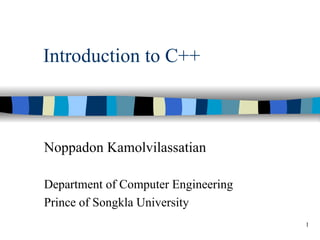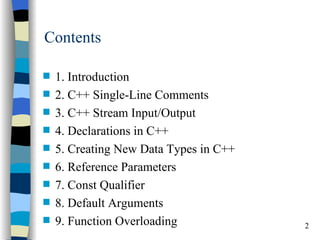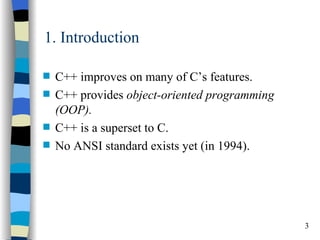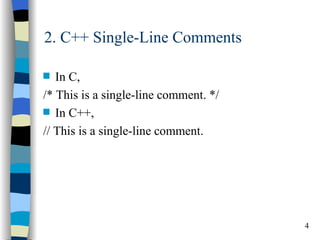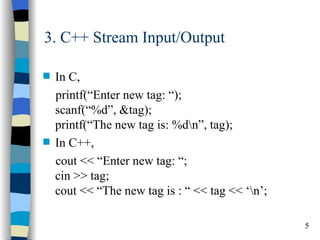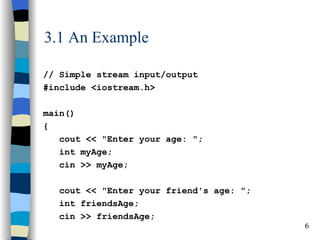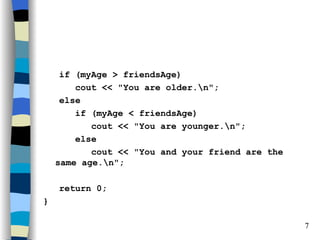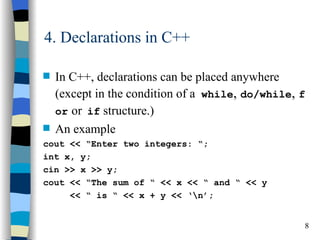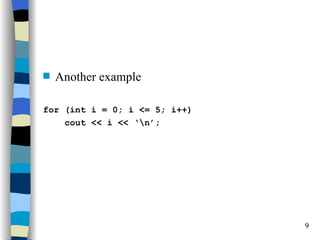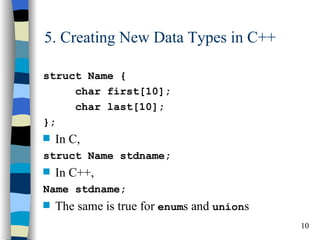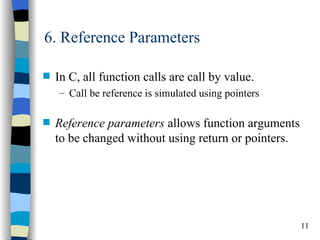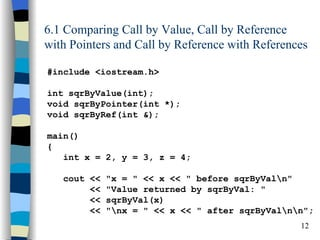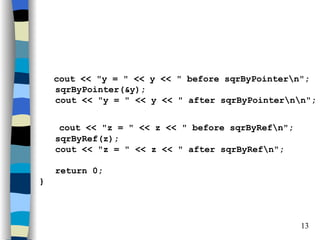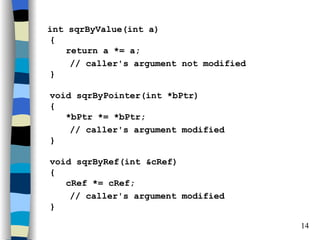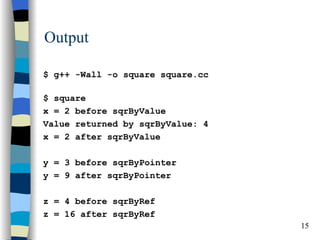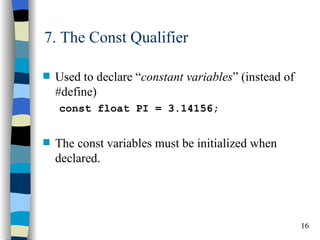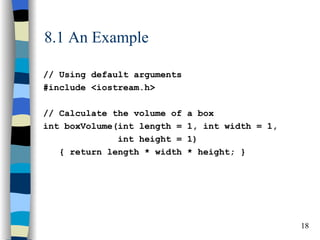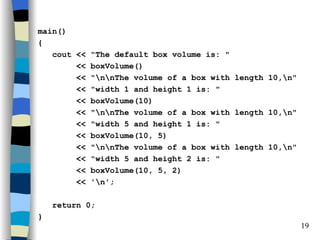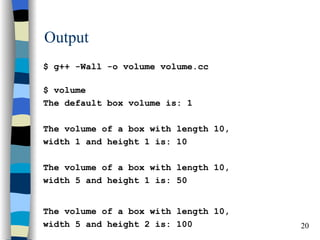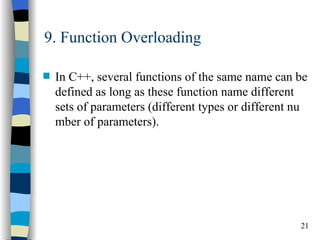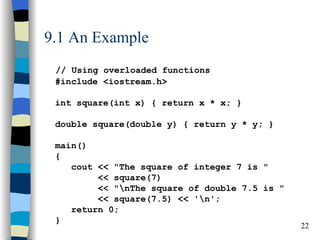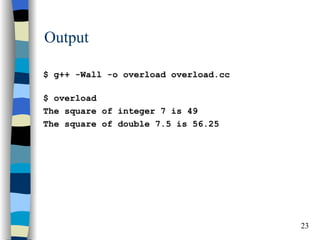1 sur 23
Publicité

### Oop1

1. Introduction to C++ Noppadon Kamolvilassatian Department of Computer Engineering Prince of Songkla University 1
2. Contents s 1. Introduction s 2. C++ Single-Line Comments s 3. C++ Stream Input/Output s 4. Declarations in C++ s 5. Creating New Data Types in C++ s 6. Reference Parameters s 7. Const Qualifier s 8. Default Arguments s 9. Function Overloading 2
3. 1. Introduction s C++ improves on many of C’s features. s C++ provides object-oriented programming (OOP). s C++ is a superset to C. s No ANSI standard exists yet (in 1994). 3
4. 2. C++ Single-Line Comments s In C, /* This is a single-line comment. */ s In C++, // This is a single-line comment. 4
5. 3. C++ Stream Input/Output s In C, printf(“Enter new tag: “); scanf(“%d”, &tag); printf(“The new tag is: %dn”, tag); s In C++, cout << “Enter new tag: “; cin >> tag; cout << “The new tag is : “ << tag << ‘n’; 5
6. 3.1 An Example // Simple stream input/output #include <iostream.h> main() { cout << "Enter your age: "; int myAge; cin >> myAge; cout << "Enter your friend's age: "; int friendsAge; cin >> friendsAge; 6
7. if (myAge > friendsAge) cout << "You are older.n"; else if (myAge < friendsAge) cout << "You are younger.n"; else cout << "You and your friend are the same age.n"; return 0; } 7
8. 4. Declarations in C++ s In C++, declarations can be placed anywhere (except in the condition of a while, do/while, f or or if structure.) s An example cout << “Enter two integers: “; int x, y; cin >> x >> y; cout << “The sum of “ << x << “ and “ << y << “ is “ << x + y << ‘n’; 8
9. s Another example for (int i = 0; i <= 5; i++) cout << i << ‘n’; 9
10. 5. Creating New Data Types in C++ struct Name { char first; char last; }; s In C, struct Name stdname; s In C++, Name stdname; s The same is true for enums and unions 10
11. 6. Reference Parameters s In C, all function calls are call by value. – Call be reference is simulated using pointers s Reference parameters allows function arguments to be changed without using return or pointers. 11
12. 6.1 Comparing Call by Value, Call by Reference with Pointers and Call by Reference with References #include <iostream.h> int sqrByValue(int); void sqrByPointer(int *); void sqrByRef(int &); main() { int x = 2, y = 3, z = 4; cout << "x = " << x << " before sqrByValn" << "Value returned by sqrByVal: " << sqrByVal(x) << "nx = " << x << " after sqrByValnn"; 12
13. cout << "y = " << y << " before sqrByPointern"; sqrByPointer(&y); cout << "y = " << y << " after sqrByPointernn"; cout << "z = " << z << " before sqrByRefn"; sqrByRef(z); cout << "z = " << z << " after sqrByRefn"; return 0; } 13
14. int sqrByValue(int a) { return a *= a; // caller's argument not modified } void sqrByPointer(int *bPtr) { *bPtr *= *bPtr; // caller's argument modified } void sqrByRef(int &cRef) { cRef *= cRef; // caller's argument modified } 14
15. Output \$ g++ -Wall -o square square.cc \$ square x = 2 before sqrByValue Value returned by sqrByValue: 4 x = 2 after sqrByValue y = 3 before sqrByPointer y = 9 after sqrByPointer z = 4 before sqrByRef z = 16 after sqrByRef 15
16. 7. The Const Qualifier s Used to declare “constant variables” (instead of #define) const float PI = 3.14156; s The const variables must be initialized when declared. 16
17. 8. Default Arguments s When a default argument is omitted in a function call, the default value of that argument is automati cally passed in the call. s Default arguments must be the rightmost (trailing) arguments. 17
18. 8.1 An Example // Using default arguments #include <iostream.h> // Calculate the volume of a box int boxVolume(int length = 1, int width = 1, int height = 1) { return length * width * height; } 18
19. main() { cout << "The default box volume is: " << boxVolume() << "nnThe volume of a box with length 10,n" << "width 1 and height 1 is: " << boxVolume(10) << "nnThe volume of a box with length 10,n" << "width 5 and height 1 is: " << boxVolume(10, 5) << "nnThe volume of a box with length 10,n" << "width 5 and height 2 is: " << boxVolume(10, 5, 2) << 'n'; return 0; } 19
20. Output \$ g++ -Wall -o volume volume.cc \$ volume The default box volume is: 1 The volume of a box with length 10, width 1 and height 1 is: 10 The volume of a box with length 10, width 5 and height 1 is: 50 The volume of a box with length 10, width 5 and height 2 is: 100 20
21. 9. Function Overloading s In C++, several functions of the same name can be defined as long as these function name different sets of parameters (different types or different nu mber of parameters). 21
22. 9.1 An Example // Using overloaded functions #include <iostream.h> int square(int x) { return x * x; } double square(double y) { return y * y; } main() { cout << "The square of integer 7 is " << square(7) << "nThe square of double 7.5 is " << square(7.5) << 'n'; return 0; } 22
23. Output \$ g++ -Wall -o overload overload.cc \$ overload The square of integer 7 is 49 The square of double 7.5 is 56.25 23
Publicité